# Get help with circle geometry problemsRecent questions in Circles
Circles
ANSWERED### Find the overlapping area of two equations also give its geometrical representation: $$\displaystyle{u}^{{{2}}}+{v}^{{{2}}}={n}{\quad\text{and}\quad}{u}^{{{2}}}+{\left({v}-{n}\right)}^{{{2}}}={1}$$ put $$\displaystyle{n}={516}$$

Circles
ANSWERED### Let D be the region between the circles of radius 6 and radius 8 centered at the origin that lies in the third quadrant. Express D in polar coordinates. Select the correct answer below: 1) $$\displaystyle{D}={\left\lbrace{\left({r},\theta\right)}{\mid}{6}\leq{r}\leq{8},{0}\leq\theta\leq{\frac{{\pi}}{{{2}}}}\right\rbrace}$$ 2) $$\displaystyle{D}={\left\lbrace\begin{array}{cc} {r}&\theta\end{array}\right)}{\mid}{6}\leq{r}\leq{8},\pi\leq\theta\leq{\frac{{{3}\pi}}{{{2}}}}\rbrace$$ 3) $$\displaystyle{D}={\left\lbrace{\left({r},\theta\right)}{\mid}{0}\leq{r}\leq{8},\pi\leq\theta\leq{\frac{{{3}\pi}}{{{2}}}}\right\rbrace}$$ 4) $$\displaystyle{D}={\left\lbrace{\left({r},\theta\right)}{\mid}{6}\leq{r}\leq{8},\pi\leq\theta\leq{2}\pi\right\rbrace}$$

Circles
ANSWERED### The graph of a polar equation is given. Select the polar equation for graph from the following options. $$\displaystyle{r}={2}{\sin{\theta}},{r}={2}{\cos{\theta}},{r}={1}+{\sin{\theta}}$$ $$\displaystyle{r}={1}-{\sin{\theta}},{r}={3}{\sin{{2}}}\theta,{r}={3}{\sin{{3}}}\theta$$

Circles
ANSWERED###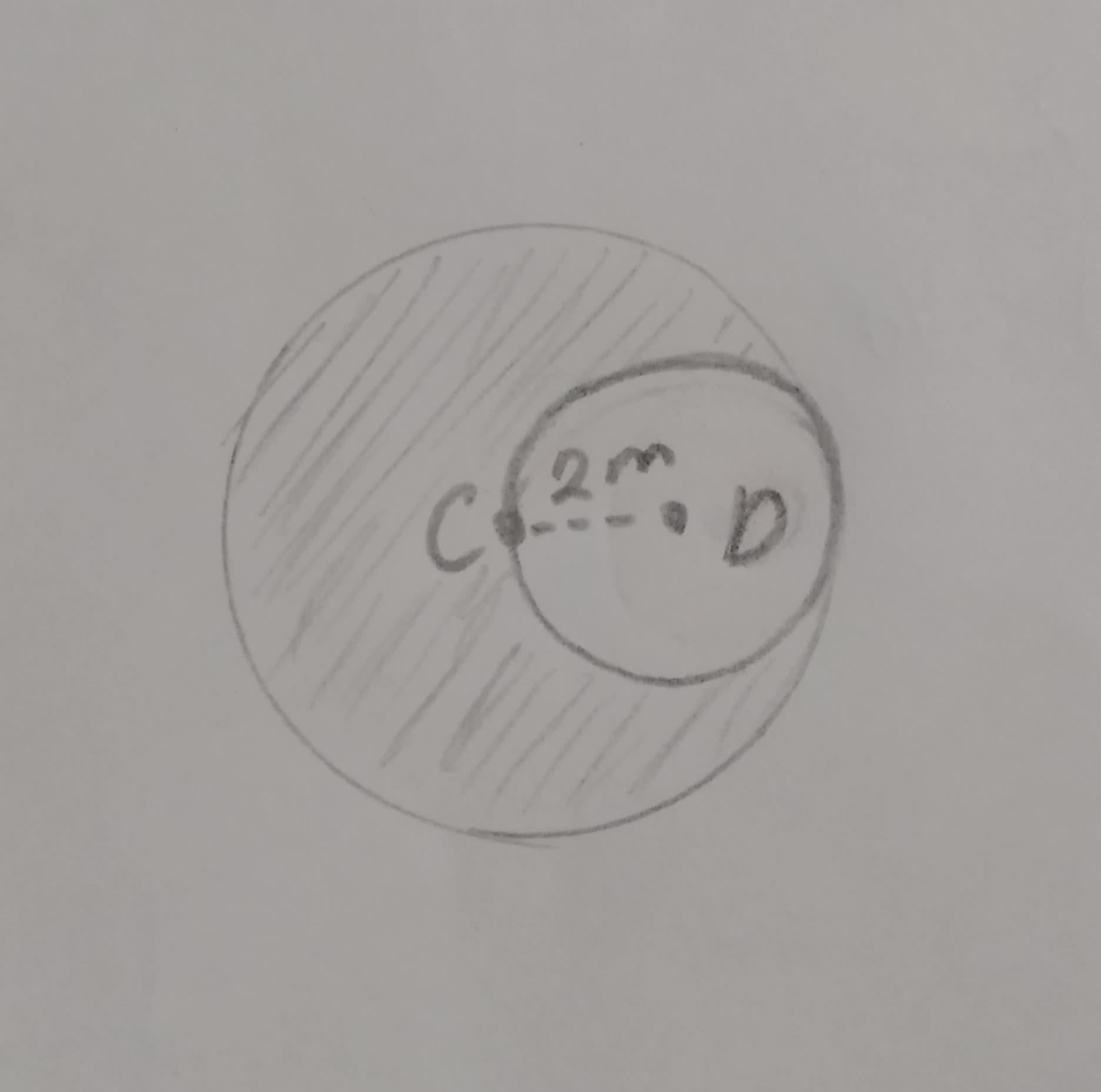Find the area of the shaded region if C and D are center

Circles
ANSWERED### Use a double integral to find the area of the region. The region inside the circle $$\displaystyle{\left({x}-{1}\right)}^{{2}}+{y}^{{2}}={1}$$ and outside the circle $$\displaystyle{x}^{{2}}+{y}^{{2}}={1}$$

Circles
ANSWERED### What is the radius and center for $$\displaystyle{\left({x}-{2}\right)}^{{{2}}}+{y}^{{{2}}}={16}?$$ A. $$\displaystyle{r}={16}$$; center is (2,0) B. $$\displaystyle{r}={4}$$; center is (-2,0) C. $$\displaystyle{r}={16}$$; center is (-2,0) D. $$\displaystyle{r}={4}$$; center is (2,0)

Circles
ANSWERED### Describe in words the surface whose equation is given. r = 5

Circles
ANSWERED### A plane passes through the common center of two concentric spheres. Describe the intersection of the plane and the two spheres.

Circles
ANSWERED### If the atomic radius of lead is 0.175 nm, calculate the volume of its unit cell in cubic meters

Circles
ANSWERED### For the region R below, write $$\displaystyle\int\int_{{Z}}{f}{d}{A}$$ as an integral in polar coordinates. ">19610601451.jpg"> Use t for $$\displaystyle\theta$$ in your expressions.

Circles
ANSWERED### Find the probability of landing in the shaded region of the figure. Rectangle with 2 inscribed circles. Length of the rectangle is 20m and the width is 10m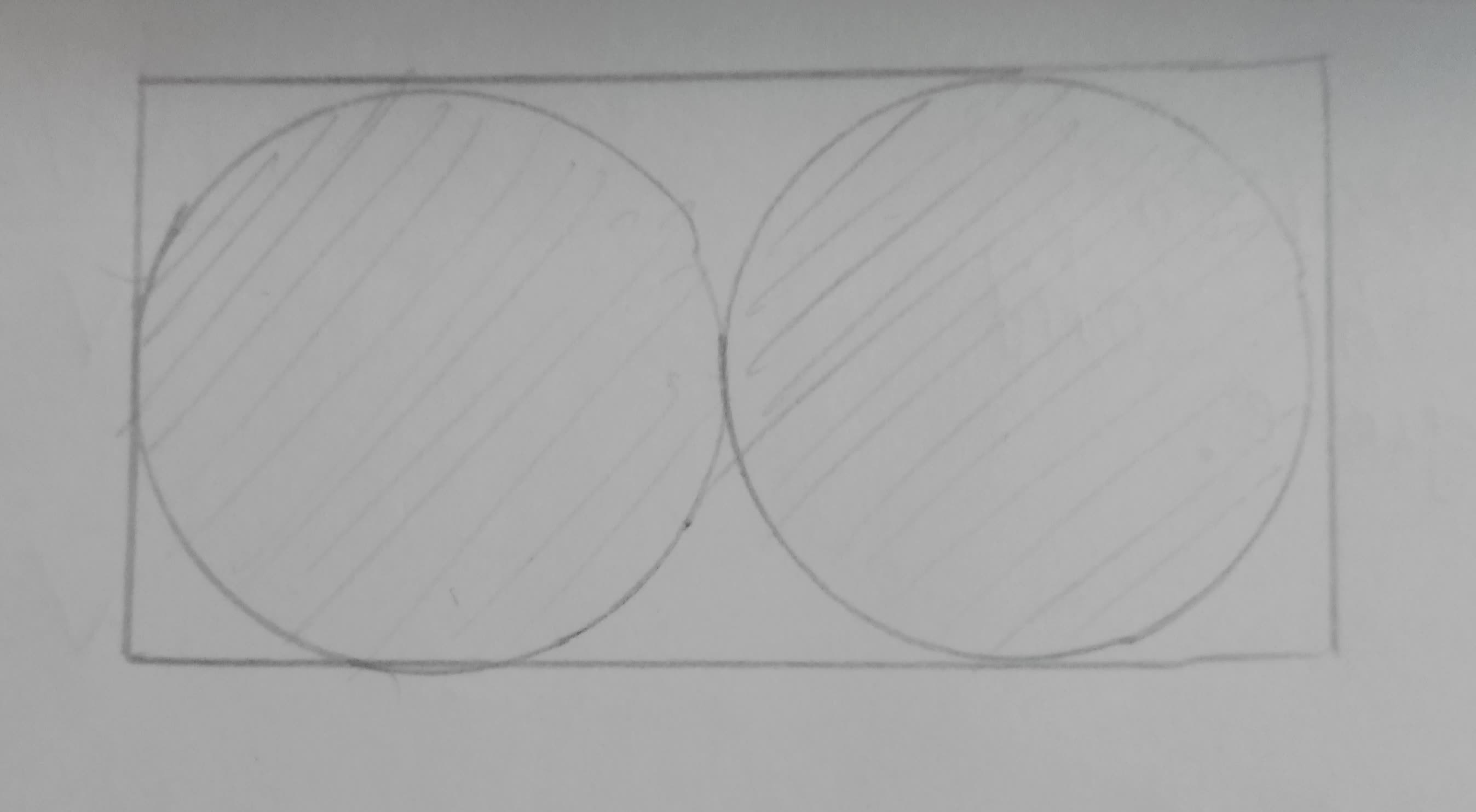Circles
ANSWERED### Find the radius of a circle whose area equals the sum of the areas of two circles with radii 3 in. and 4 in. respectively.

Circles
ANSWERED### Find the differential equation of all circles passing through the origin and having their centers on the x-axis.

Circles
ANSWERED### Write the equation of a line passing through the centers of the two circles $$\displaystyle{\left({x}-{3}\right)}^{{{2}}}+{\left({y}+{2}\right)}^{{{2}}}={16}$$ and $$\displaystyle{\left({x}-{6}\right)}^{{{2}}}+{\left({y}+{1}\right)}^{{{2}}}={25}$$

Circles
ANSWERED### To construct: The angle equal to the average of measures of $$\displaystyle\angle{A}$$ and $$\displaystyle\angle{B}$$.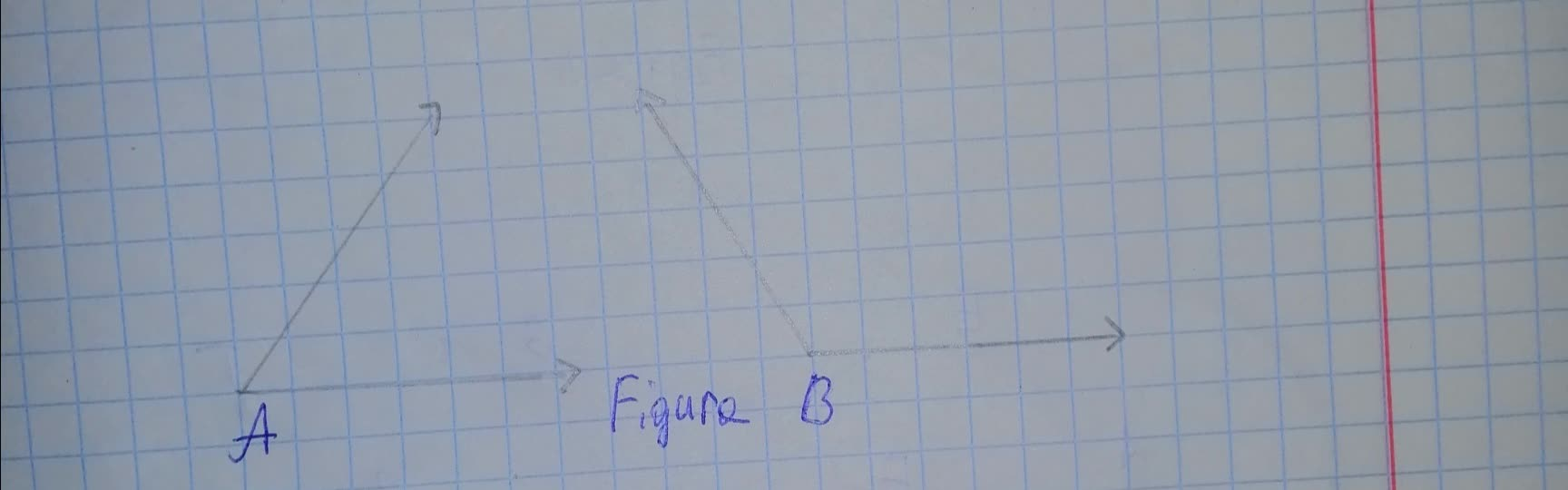Circles
ANSWERED### The measure to be used to prove that all hoops are congruent. Given: A fitness instructor wants to confirm that all the hoops are of same size.

Circles
ANSWERED### A Geometric Model: The ancient Greeks often discovered mathematical relationships by using geometric drawings. Study the accompanying drawing and use inductive reasoning to determine what needs to be put in place of the question mark the equation a true statement.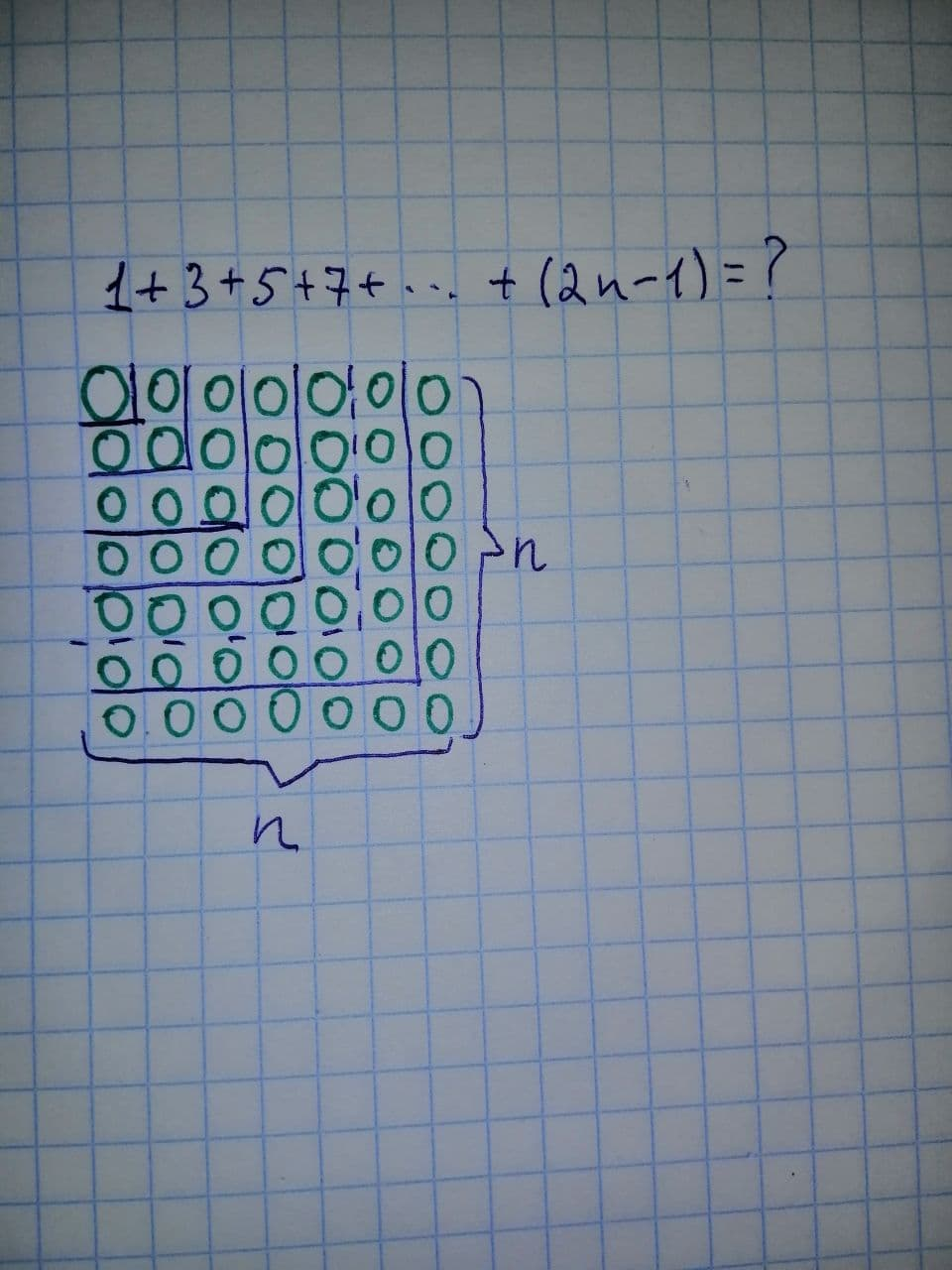How does the sum of the first odd integers related to the geometric model? Is it possible to find the sum of the first even integers using geometric model? Why or why not?

Circles
ANSWERED### For the two circles in Figures (a), (b), and (c), find the total number of common tangents (internal and external). a)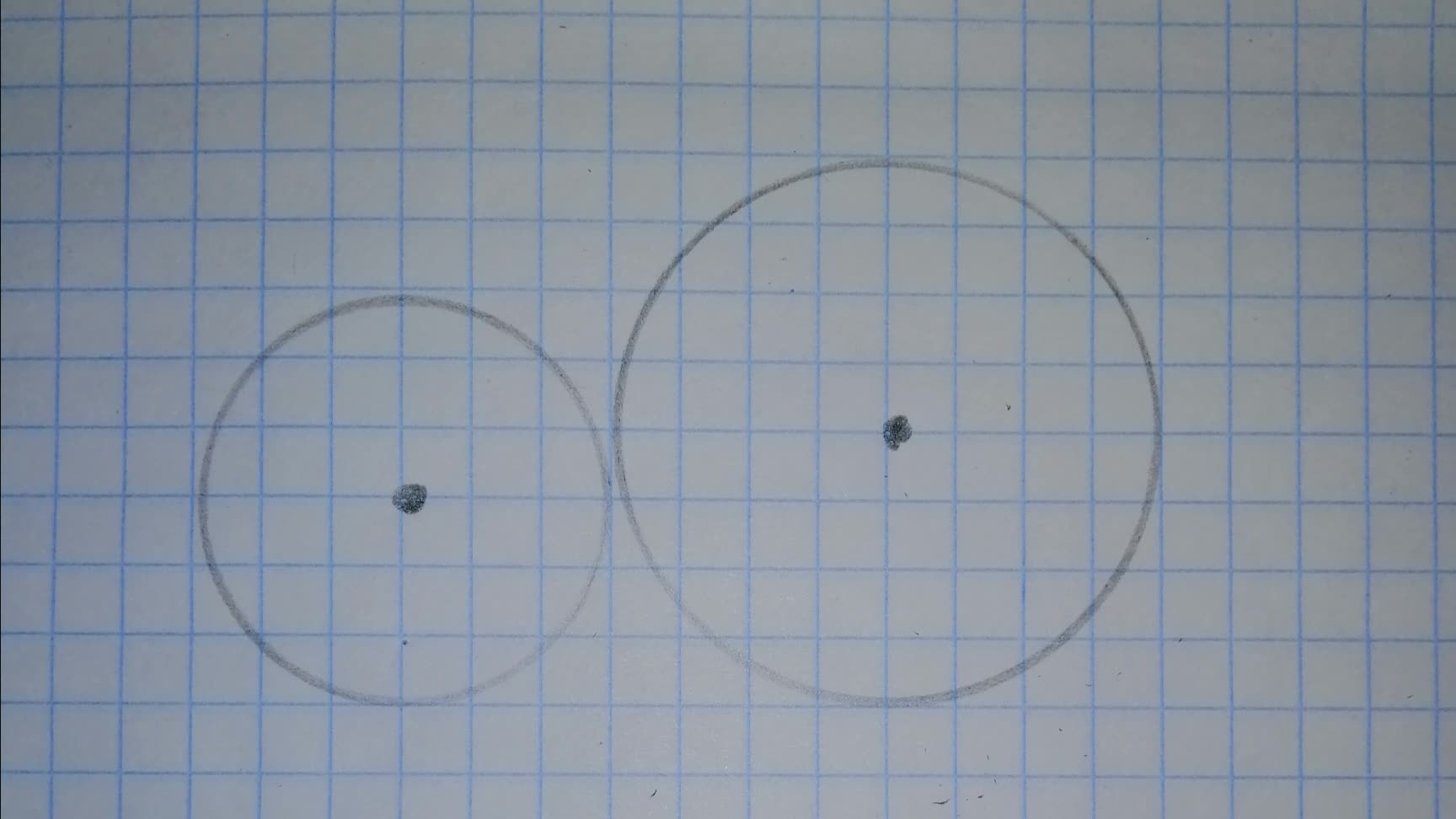b)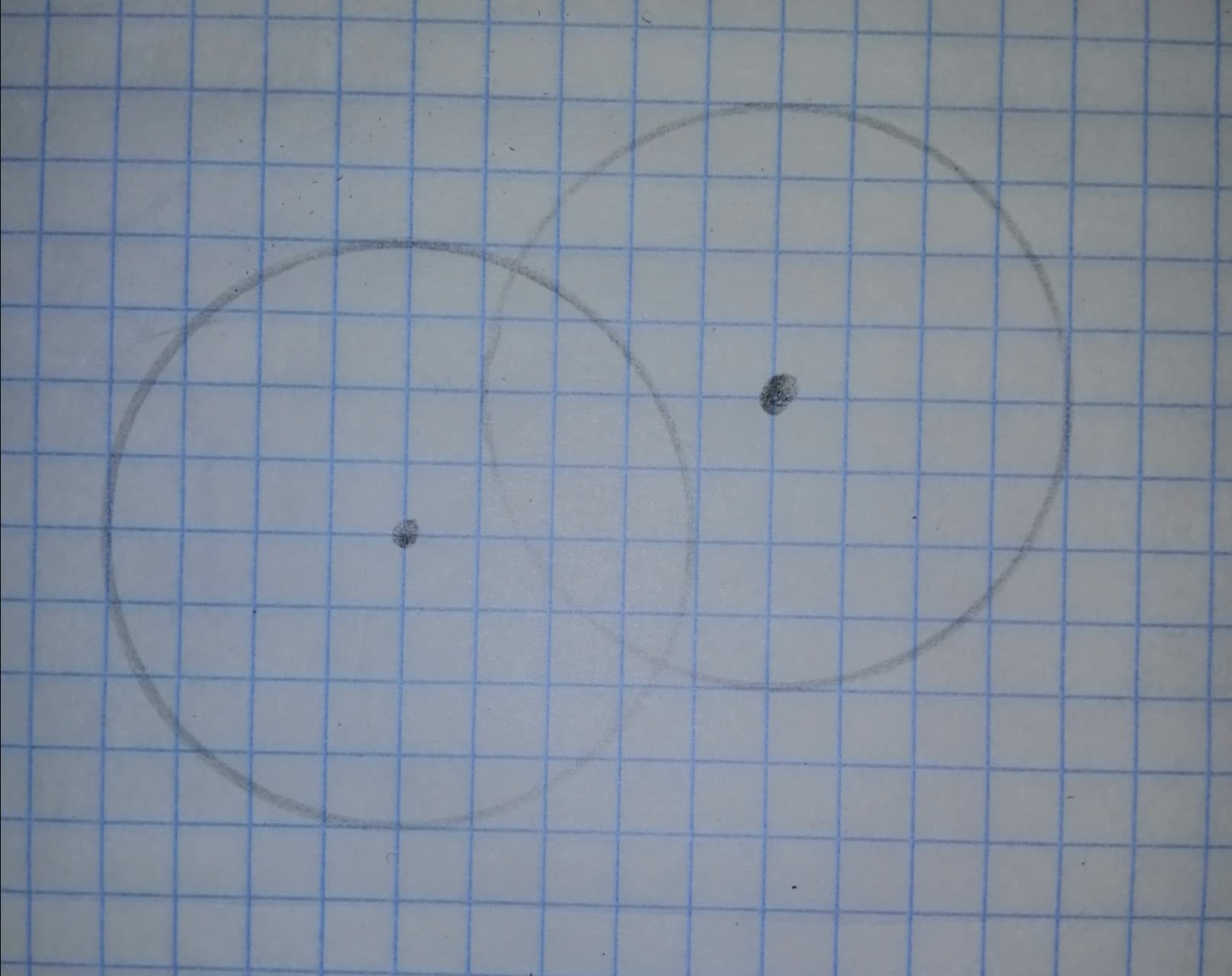c)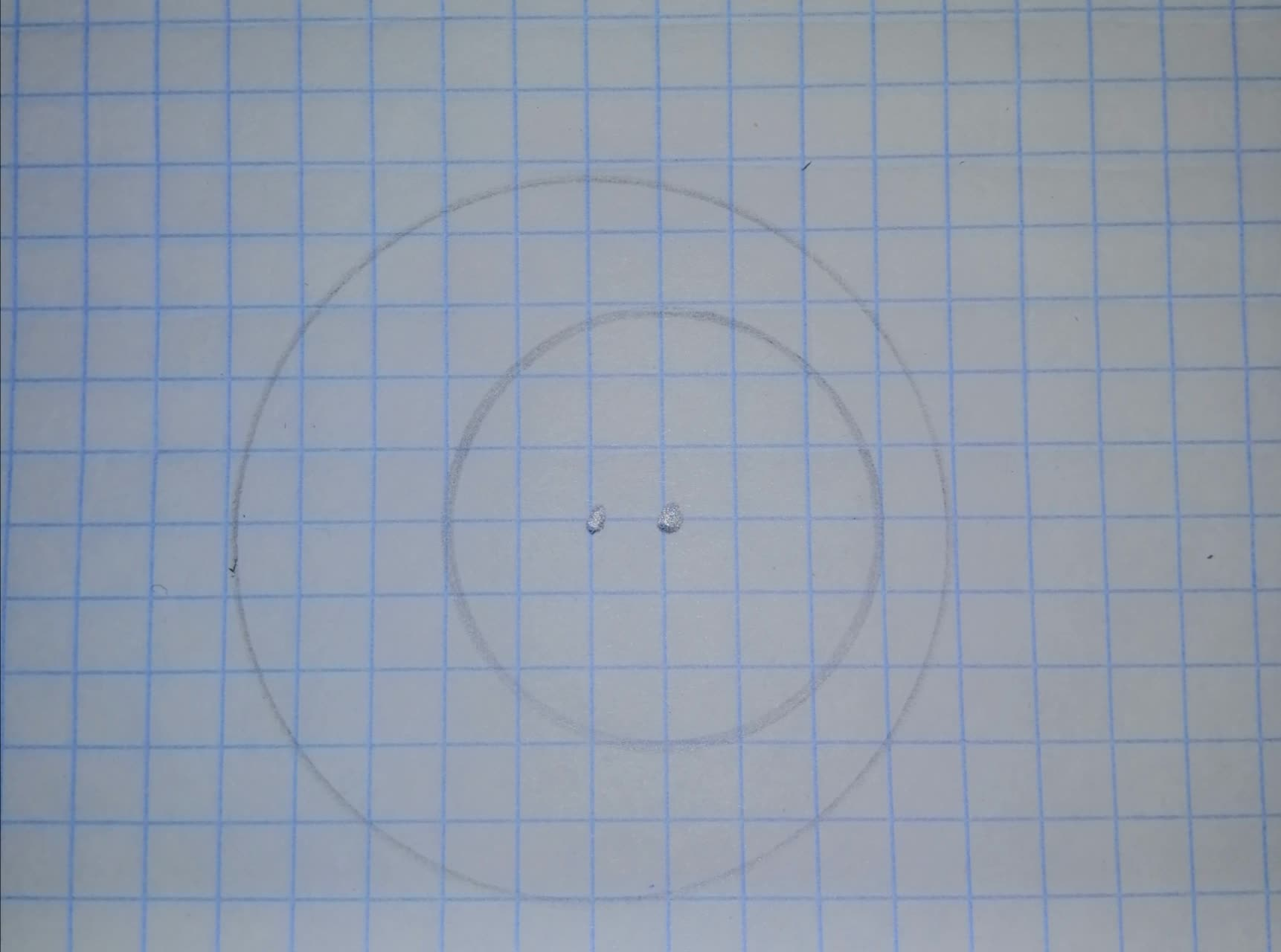Circles
ANSWERED### Write the equation of a line passing through the centers of the two circles $$\displaystyle{\left({x}-{3}\right)}^{{{2}}}+{\left({y}+{2}\right)}^{{{2}}}={16}$$ and $$\displaystyle{\left({x}-{6}\right)}^{{{2}}}+{\left({y}+{1}\right)}^{{{2}}}={25}$$
ANSWERED